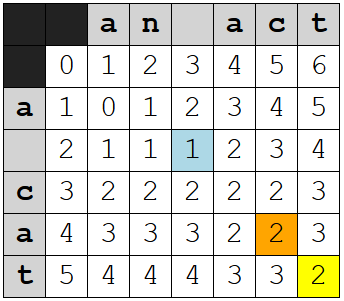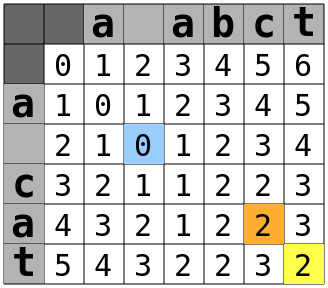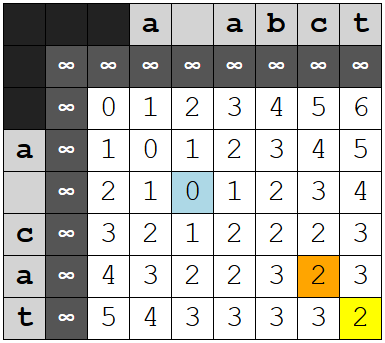# Damerau-Levenshtein Edit Distance Explained

By James M. Jensen II, Sunday, April 7, 2013

For my master's studio, I implemented the Wagner-Fischer algorithm for finding the Levenshtein edit distance between two protein sequences to find the closest match from a database of protein sequences to an input sequence. It's a very elegant algorithm, and has a straightforward explanation that can be easily found with a quick web search.

Now, Wagner-Fischer has a cousin, the algorithm for calculating the Damerau-Levenshtein edit distance, which does not have nearly as many resources available to those wanting to learn it. The pseudocode on Wikipedia is not very expressive. I couldn't find a good explanation of how it works. So what I did was implemented the pseudocode in Python and walked through it, renaming the confusing variables as I figured out their role. You can find my Python code here. (I've also implemented it in C99.)

## First Steps: Restricted Edit Distance

To understand Damerau-Levenshtein, it helps to to understand a simple variant on Wagner-Fischer for calculating the "restricted edit distance."

The change is very small: at each step, in addition to calculating the cost of an addition, a deletion, and a substitution, and taking the lowest, you also check for a transposition: if the last two elements in each sequence are the same but swapped, then you look back two columns and rows to find the cost from before the transposition, then add one to that. If that's the lowest value, then that's what you use.

For example, take the case of the strings A = "a cat" and B = "an act." The Levenshtein distance for this is 3: to get from A to B requires one addition (the 'n') and two substitutions ('a' to 'c' and 'c' to 'a').

The Wagner-Fischer table ends up looking like this:

I've highlighted the last cell, which gives us the total cost.

With the addition of the check for transpositions, though, the algorithm can notice that "ac" and "ca" are swapped, not substituted, and you get this table instead:Table for calculating restricted edit distance between "a cat" and "an act"

I've highlighted the relevant cells:

• The last cell, of course, which now has a value of 2
• The cell at ("a", "c") that also has a value of 2 because the algorithm recognizes the transposition
• The cell at the intersection of the spaces, which is the basis of the cost for the cell at ("a", "c")

At ("a", "c"), the cost of a transposition was calculated by looking backward to the cell at the intersections of the spaces, which represents the total cost before the transposition, and adding one to it. Essentially, we're rewinding to the point before the transposition and checking to see if that gives us a smaller cost.

## Limitations of Restricted Edit Distance

So far so good. For most applications, my guess is restricted edit distance is as far as you really need to go: it's simple and it can be optimized for memory usage like Wagner-Fischer. The only difference is you need to keep three rows in memory at any given time, instead of two.

Restricted edit distance makes an assumption that causes some problems in some cases, though: it assumes no characters were added or deleted between the transposed characters. This means that "a cat" and "an abct" will have an edit distance of 4, even though if you work it out on paper you can get from the first to the second in three moves:

Similarly, from "a cat" to "a tc" is a distance of 3 for restricted edit distance but you can get there in two moves on paper:

 a cat Deletion: a ct Transposition: a tc

This may seem like a minor problem, but a consequence of it is that the triangle inequality does not hold for restricted edit distance: the distance between two sequences can be greater than the sum of the distances between each to a common third sequence. It's like being told it's a shorter walk to the grocery store if you go to the other side of town first than it is to walk there directly.

## Damerau-Levenshtein

This brings us (finally) to Damerau-Levenshtein, which does not have the limitations of restricted edit distance.

The main difference between Damarau-Levenshtein and the reduced edit distance algorithm is that when Damerau-Levenshtein computes a transposition it will generally look much further backwards to find a match than the reduced edit distance algorithm will. Specifically, it tries to find:

• The last row with the current column's character
• The last column in this row where the characters matched

If these exist, they will together define a cell: call that C1. It then looks back to the cell to the upper-left of that one: call that C2. You can think of C2 as the total at an earlier state in the process, the state just before the transposition. If there's a transposition, the system in a way reverts to that earlier state, then calculates the cost of getting back to where we were given that it now knows there's a transposition between here and there.

Of course, we don't actually rewrite any of the other table cells: that would be a Bad Thing™, since any cell (r,c) in the table should be the correct edit distance for the subsequences A[0..r] and B[0..c]. It's even possible, I think, that the system might decide later that it was wrong about the transposition.

So how do we redo the work without actually redoing it? Thankfully, the assumption that it's either all additions or all deletions allows us to simply count the number of characters in between the transposed characters, in either A or B. If they're deletions, we count on A. If they're additions, we count on B.

And by "count," of course, I really mean "subtract the lower row/col number from the higher one," minus one to make it exclude the current cell from the count.

Here's more good news: due to the fact that the value of the cell will be the lowest of the costs of addition, deletion, substitution, or transposition, we don't actually have to check whether the differences in the middle of a transposition are additions or deletions: we just count both directions, and if neither is zero, the cost will simply be too high for that cost to be chosen. (Thanks go to Steve at Programmers Stack Exchange for helping me realize this.)

Thus, the cost of a transposition is calculated as
(cost before transposition) + (distance between rows) + (distance between columns) + 1

In both my Python and my C versions this is implemented as:

```    matrix[last_matching_row][last_match_col]
+ (row - last_matching_row - 1) + 1
+ (col - last_match_col - 1))
```

(Note that `last_matching_row` and `last_match_col` are each one lower than the row/col they actually represent. This was in the original pseudocode and makes some of the logic a little more elegant.)

Now that we have the costs for addition, deletion, substitution, and transposition, we just set the current cell to whichever value is lowest. Here's the table for "a cat" to "a abct":Table for Damerau-Levenshtein edit distance for "a cat" to "a abct"

When the process gets to the cell highlighted in orange, it looks backwards and finds that the last match that row was two columns beforehand, in column 3, and the last row corresponding to a "c" was one row behind, in row 3. The cell to the upper-left of that cell is (2,2), highlighted in blue. That cell has a value of zero. Add in one for the one character added between the transposed characters, and one for the transposition itself and you get 0 + 1 + 1 = 2.

And that's how Damarau-Levenshtein works.

## Appendix: Implementation Notes

There are a couple of differences worth mentioning between how I just described things and how they are implemented in my code and the original pseudocode.

The first is that in the code, we don't actually look backward to find a matching column and row for a transposition. Instead, we keep a map, last_row, holding the number of the last row where a character was seen in A, and a variable last_match_col that holds the column number of the last match in that row. These are updated at the end of each iteration of either the inner or outer for loop.

The second was alluded to in my code for calculating the transposition cost, above: the last_matching_row and last_match_col are one lower than they should be. Actually, it's the table itself that's one row and column longer than it should be.

There is an extra row and column in the table. The topmost row and the leftmost column are filled with a large number, one denoted INF for "infinity," a number higher than the highest possible distance for the pair. Thus, the actual table for the above pair would look like this:Table for Damarau-Levenshtein for "a cat" to "abct" (with infinity row and column)

The purpose of this is to simplify the logic somewhat. This way, we can initialize the values for all entries in last_row (not done in Python, where I use a dictionary, but this is the way I did it in C) and the value of last_match_row both to zero. Zero in this context means "no match," and any attempt to calculate a transposition where either last_matching_row (which is drawn for last_row) or last_match_col is zero will return a cost far too high to ever be chosen as the minimum.

That's all, folks!

 Note: in most implementations, doubled elements like "aa," which technically meet the criteria for a transposition - each being equal to the other - are not detected explicitly. Instead it's handled the same way matches are for substitutions: the additional cost is one if the characters are different and zero if they're the same.

 In the real world, of course, it's conceivable that the long way might be faster, due to traffic or obstructions or whatnot along the short route, but it will never be shorter.

 In the code INF is defined as the sum of the lengths of the sequences. The maximum edit distance for any pair of sequences is the length of the longer of the two - it can't get worse than substituting all characters in the smaller string and adding in the remainder from the longer - so any value larger than that is effectively infinite as far as this algorithm is concern. Adding the two lengths together is the quickest way to ensure we get a sufficiently large number.

### References

1. Damerau, Fred J. (March 1964), "A technique for computer detection and correction of spelling errors", Communications of the ACM, ACM, 7 (3): 171–176, doi:10.1145/363958.363994
2. R. Wagner and M. Fischer (1974), "The string to string correction problem", Journal of the ACM, 21:168-178, doi:10.1145/321796.321811